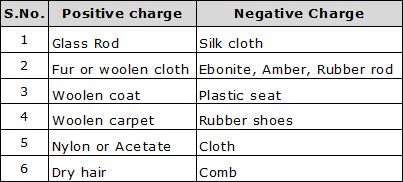# ELECTRIC CHARGE

(a)    Definition: Electric charge may be defined as the intrinsic property of certain fundamental particles (electron, proton, etc) due to which they produce electric and magnetic effects.

(B)    Charge on a Macrobody: Excess or deficiency of electrons in a body is equal to the charge on a macrobody. A body having excess of electrons in negatively charge and a body having deficiency of electrons is positively charged.
From the study of atomic structure, we know that an atom consists of a central part called nucleus and around the nucleus there are a number of electrons revolving in different paths or orbits. The nucleus contains protons and neutrons. A proton is a positively charged particle while a neutron has no charge. Therefore, the nucleus of an atom bears a positive charge. An electron is a negatively charged particle having negative charge equal to the positive charge on a proton. Normally, the number of electrons is equal to the number of protons in an atom. Therefore, an atom is neutral as a whole; the negative charge on electrons cancelling the positive charge on protons. This leads to the conclusion that under ordinary conditions, a body is neutral i.e. it exhibits no charge.
When this equity or balance is disturbed by removing or supplying electrons, the body acquires a net charge. The body will acquire a positive or negative charge depending upon whether electrons are removed from it or added to it.

(C)    Types of Electric Charge: There are two types of charges. They are:
(i) Positive charge: A body having deficiency of electrons.
(ii) Negative charge: A body having excess of electrons.

(D)    Charging of a Body: There are a number of methods to charge a body as:
(i) Charging by friction
(ii) Charging by conduction
(iii) Charging by induction etc.
We will discuss charging by friction in detail:
Whenever two bodies (at least one non conductor) are rubbed against each other, heat is produced due to friction present between them. Due to this heat produced, electrons in both the bodies are excited. The body having more electron affinity attracts some of the electrons from other body. Both the bodies develop equal and opposite charges by this method.(E)    Properties of Electric Charge:
(i) Like charges repel and unlike charges attract each other.
(ii) Charge is a scalar quantity.
(iii) Charge is always quantized.
(iv) Charge is conserved.
(v) Charge is always associated with mass.

(F)    Unit of Charge: The charge on an electron is so small that it is not convenient to select it the unit of charge. In practice, coulomb is used as the unit of charge, i.e. SI unit of charge is coulomb abbreviated as C. One coulomb of charge is equal to the charge on 625 × 1016 electrons.
1 coulomb = charge on 625 × 1016 electrons or